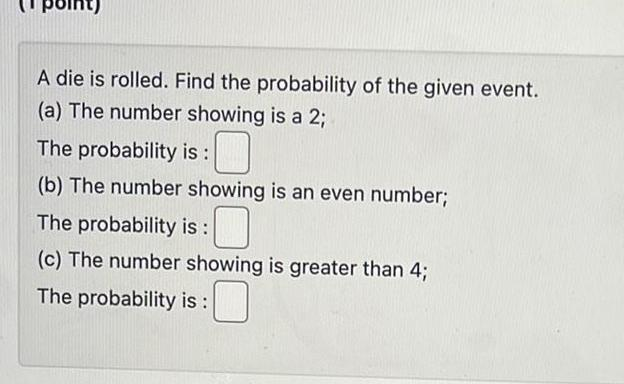Question:

# A die is rolled Find the probability of the given event a

Last updated: 9/18/2023A die is rolled Find the probability of the given event a The number showing is a 2 The probability is b The number showing is an even number The probability is c The number showing is greater than 4 The probability is